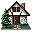Home Existing users log in here or new users sign up here. Nature of Motion Types of Motion Galileo's Pisa Experiment Projectile Motion Juggling

### Glider on Air Track: Quantitative Observational Experiment

#### Aim

To determine how the position of three different gliders depend on the clock reading. (Notice that the air glider is used in this experiment, its interaction with the track is negligible.)

none

#### Description of the Experiment

Observe the motion of the glider for each of the three experiments. Record the position of the glider frame by frame in a table. For each experiment, represent a glider with a dot (decide which part of the glider this dot will represent) and place the dots on the paper so that their positions represent the position of the glider at each frame. Use an arrow to show the direction of motion. Then plot a position-versus-time graph putting the data from three experiments on the same graph. Consider zero clock reading for each experiment to be the same. Describe the difference among the graphs and relate this difference to the difference in the glider's motions. Decide what type of motion the glider exhibits: motion with constant rate or motion with changing rate. Decide how you can describe the difference between the motion of the glider in each experiment. Describe the difference qualitatively as you see it in the dot diagrams and graphs. Invent a physical quantity that will describe the motion of the glider in each experiment.